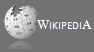Completely Solved C, C++ Programs Assignment.Quick Search Database Videos Tutorials Ebooks Forums FAQ Aboutus Household Industrial Manufacturing Service Shopping Transportation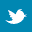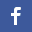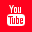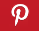### Data structure(Infix,Prefix,Postfix)-PART 3

 Filed Under: Moved to New Place

 II. INFIX, POSTFIX AND PREFIX
Now that you know what infix, prefix and postfix operations are, let us see how we can programmatically convert expressions from one form into another.
This can be done by various methods but I'll be explaining the two most common techniques:
1) USING STACKS
2) USING EXPRESSION TREES
Let us study the first technique.

 IV. A CONVERSION USING STACKS (infix to postfix expression)
In this method, we read each character one by one from the infix expression and follow a certain set of steps. The Stack is used to hold only the operators of the expression. This is required to ensure that the priority of operators is taken into consideration during conversion.
Here's the Algorithm to the Infix to Postfix Convertor Function:
Algorithm infix2postfix(infix expression string, postfix expression string)
{
char *i,*p;
i = first element in infix expression
p = first element in postfix expression
while i is not null
{
while i is a space or tab
{
increment i to next character in infix expression;
}
if( i is an operand )
{
p = i;
increment i to next character in infix expression;
increment p to next character in postfix expression;
}
if( i is '(' )
{
stack_push(i);
increment i to next character in infix expression;
}
if( i is ')' )
{
while( element at top of stack != '(' )
{
p = element at top of stack;
increment p to next character in postfix expression;
}
increment i to next character in infix expression;
}
if( i is an operator )
{
if( stack is empty )
stack_push(i);
else
{
while priority(element at top of stack) >= priority(i)
{
p = element at top of stack;
increment p to next character in postfix expression;
}
stack_push(i);
}
increment i to next character in infix expression;
}   }
while stack is not empty
{
p = stack_pop()
increment p to next character in postfix expression;
}
p = '\0';
Try converting an infix expression A + B / C to prefix on paper using the above algorithm and check if you're getting the correct result.
Now, you can see how the above algorithm is implemented in C. In the following C code, I have added a 'whitespace adder' feature to the infix2postfix() function.
If the third parameter is 0, A + B / C will be converted as ABC/+
If the third parameter is 1, A + B / C will be converted as A B C / +
Because, in actual practice 32 + 23 would be converted to 3223+. This could mean 3 + 223, 32 + 23 or 322 +3.
Hence to prevent such cases, pass 1 as the third parameter to get 32 23 +.